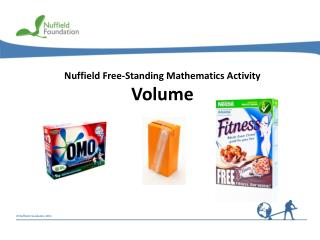DownloadDownload PresentationNuffield Free-Standing Mathematics Activity Volume

# Nuffield Free-Standing Mathematics Activity Volume

Download Presentation## Nuffield Free-Standing Mathematics Activity Volume

- - - - - - - - - - - - - - - - - - - - - - - - - - - E N D - - - - - - - - - - - - - - - - - - - - - - - - - - -
##### Presentation Transcript

1. Volume The containers for these products are all cuboids. Companies need to know how much containers like these can hold. This activity is about finding the volume of a variety of cuboids like these.

2. 1 cm 1 mm 1 mm 1 cm 1 mm 1 cm Volume The volume of a shape is the amount of space it fills. 1 m 1mm3 1 m 1 m3 1 cm3 1 m 1 cubic metre

3. 3 cm 2 cm 4 cm Volume of a cuboid Volume = 4 × 2 × 3 Volume = 24 cm3 Volume = length × width × height Volume = area of cross-section x length

4. Example 60 cm 50 cm 120 cm For a cuboid Volume = length × width × height or Volume = area of cross-section x length Volume of the fish tank Volume = 120 × 50 × 60 = 120 × 3000 cm3 = 360 000 Capacity in litres = 360 000 ÷ 1000 (1 litre = 1000 cm3) = 360 litres

5. Example Concrete block 10 cm 12 cm 2.5 m For a cuboid Volume = length × width × height or Volume = area of cross-section x length = 250 cm Think about…Why might there be a problem with these dimensions? Volume = 250 × 12 × 10 = 2500 × 12 Volume = 30 000 cm3

6. 20 cm 1.5 m 2 m For a cuboid Volume = length × width × height or Volume = area of cross-section x length Example Sand in sandpit = 0.2m Think about…Which dimension should be converted? Volume = 2 × 1.5 × 0.2 = 3 × 0.2 = 0.6 Volume = 0.6 m3

7. Volume • Reflect on your work A manufacturer needs to know the volume of a box (cuboid). Explain how to find this. What units can volume be measured in? Suggest dimensions that you could use to make a carton with a volume of 1 litre (1000 cm³).